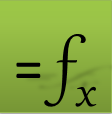## Using DYNVAL to define constraints on array results

DYNVAL is a dummy function that simply returns the value of its argument but in an optimization context, it ensures that its argument is dynamically evaluated during the optimization. In ExceLab 7.0 and later you can define arbitrary constraint formulas on the array result simply by referencing any value or range of values in the array result in your constraint formulas by using DYNVAL.

If you have used any of the pre ExceLab 7.0 criterion functions ARRAYVAL, ODEVAL or PDEVAL for imposing constraints on array results, the examples below show how to easily convert your formulas to use the new DYNVAL function in ExceLab 7.0 or later.

## Quick Examples

Let the array below represent the results of an initial value problem simulation using IVSOLVE.

 A B C 1 Time Disp Velocity 2 0 1 0 3 0.1 0.95711 -0.26427 4 0.2 0.846225 -0.4425 5 0.3 0.692986 -0.54106 6 0.4 0.520265 -0.57127 .. 41 3.9 -0.00483 0.004681 42 4.0 -0.00339 0.004634
• To constrain the value in B5 (which corresponds to the value of Disp at Time =0.3 to be 0.5.

• ExceLab 7.0 and later:
=DYNVAL(B5) - 0.5
• Pre ExceLab 7.0:
=ARRAYVAL(B5) - 0.5
• To constrain the maxium value for the Disp, column B2:B12, to be less than or equal be 0.5.

• ExceLab 7.0 and later:
=MAX(DYNVAL(B2:B12)) - 0.5
• Pre ExceLab 7.0:
=ARRAYVAL(B2:B12, "MAX") - 0.5
• To constrain the interpolated value of the Disp at Time=0.35 to be 0.6.

• ExceLab 7.0 and later:
=INTERPXY(A2:A12, DYNVAL(B2:B12), 0.35) – 0.6
• Pre ExceLab 7.0:
=ODEVAL(A2:A12, Disp, "INTERP", 0.35) – 0.6
• To constrain the derivative of the Disp at Time=0.35 to be 0.

• ExceLab 7.0 and later:
=DERIVXY(A2:A12, DYNVAL(B2:B12), 0.35) – 0
• Pre ExceLab 7.0:
=ODEVAL(A2:A12, Disp, "DERIV", 0.35) – 0
Question or Comment? Email us:
support @ excel-works.comExceLab: Transforming Excel into a Calculus Power House
ExceLab functions and methods are protected by USA Patents 9286286, 9892108, 10114812 and pending.